Next: Statistical Mechanics Up: Probability Theory Previous: Central Limit Theorem

# Exercises

1. Let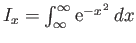and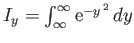. Show that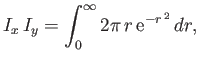where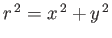. Hence, deduce that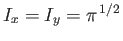.

2. Show that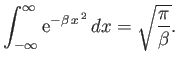Hence, deduce that3. Confirm that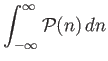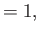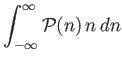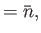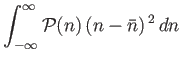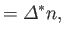where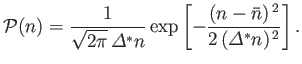4. Show that the probability of throwing 6 points or less with three (six-sided) dice is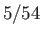.

5. Consider a game in which six (six-sided) dice are rolled. Find the probability of obtaining:
1. exactly one ace.
2. at least one ace.
3. exactly two aces.

6. In the game of Russian roulette, one inserts a single cartridge into the drum of a revolver, leaving the other five chambers of the drum empty. One then spins the drum, aims at one's head, and pulls the trigger.
1. Show that the probability of still being alive after playing the game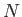times is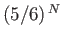.
2. Show that the probability of surviving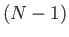turns in this game, and then being shot theth times one pulls the trigger, is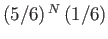.
3. Show that the mean number of times a player gets to pull the trigger is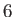.

7. A battery of total emfis connected to a resistor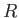. As a result, an amount of power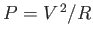is dissipated in the resistor. The battery itself consists ofindividual cells connected in series, so thatis equal to the sum of the emf's of all these cells. The battery is old, however, so that not all cells are in perfect condition. Thus, there is a probabilitythat the emf of any individual cell has its normal value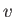; and a probability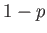that the emf of any individual cell is zero because the cell has become internally shorted. The individual cells are statistically independent of each other. Under these conditions, show that the mean power,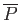, dissipated in the resistor, is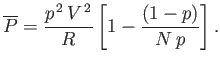8. A drunk starts out from a lamppost in the middle of a street, taking steps of uniform length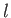to the right or to the left with equal probability.
1. Show that the average distance from the lamppost aftersteps is zero.
2. Show that the root-mean-square distance (i.e. the square-root of the mean of the distance squared) from the lamppost aftersteps is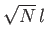.
3. Show that the probability that the drunk will return to the lamppost aftersteps is zero ifis odd, and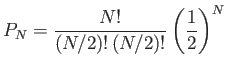ifis even.

9. A molecule in a gas moves equal distancesbetween collisions with equal probabilities in any direction. Show that, after a total ofsuch displacements, the mean-square displacement,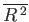, of the molecule from its starting point is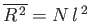.

10. A penny is tossed 400 times. Find the probability of getting 215 heads. (Use the Gaussian distribution.)

11. Suppose that the probability density for the speed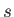of a car on a highway is given by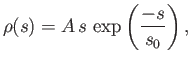where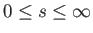. Here,and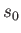are positive constants. More explicitly,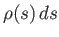gives the probability that a car has a speed betweenand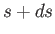.
1. Determinein terms of.
2. What is the mean value of the speed?
3. What is the most probable'' speed: that is, the speed for which the probability density has a maximum.
4. What is the probability that a car has a speed more than three times as large as the mean value?Next: Statistical Mechanics Up: Probability Theory Previous: Central Limit Theorem
Richard Fitzpatrick 2016-01-25